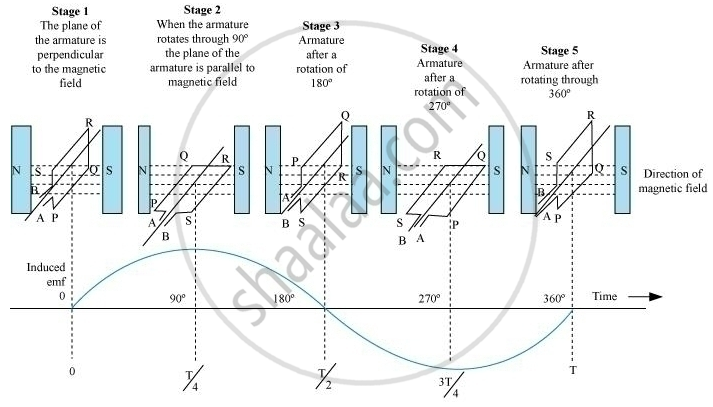# Show Diagrammatically How an Alternating Emf is Generated by a Loop of Wire Rotating in a Magnetic Field. Write the Expression for the Instantaneous Value of the Emf Induced in the Rotating Loop. - Physics

#### Question

Show diagrammatically how an alternating emf is generated by a loop of wire rotating in a magnetic field. Write the expression for the instantaneous value of the emf induced in the rotating loop.

#### Solution

Generation of an alternating e.m.f. by a loop of wire rotating in a magnetic fieldExpression of the instantaneous value of the induced e.m.f. in a rotating loop

ε = NBAω sinωt

Where N = number of turns in armature coil

= Magnetic field vector

=Area vector of the coil

ω Angular speed

Is there an error in this question or solution?

#### Video TutorialsVIEW ALL 

Show Diagrammatically How an Alternating Emf is Generated by a Loop of Wire Rotating in a Magnetic Field. Write the Expression for the Instantaneous Value of the Emf Induced in the Rotating Loop. Concept: Electromagnetic Induction.